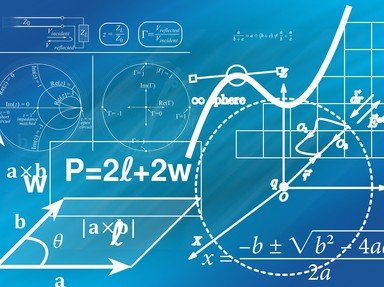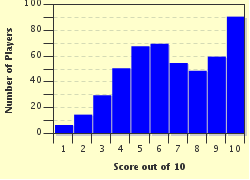# Fibonacci Numbers Trivia Quiz

### Fibonacci numbers are claimed to be the nature's numbering system. How much do you know about them? Have fun and thanks for playing.

A multiple-choice quiz by Matthew_07. Estimated time: 4 mins.

Author
Matthew_07
Time
4 mins
Type
Multiple Choice
Quiz #
266,099
Updated
Dec 03 21
# Qns
10
Difficulty
Average
Avg Score
6 / 10
Plays
2478
Awards
Top 35% Quiz
Last 3 plays: Pnch (6/10), Guest 136 (5/10), Guest 112 (7/10).
1. The first few Fibonacci numbers are 0, 1, 1, 2, 3, 5, 8, 13... These numbers are named after Fibonacci, whose real name is? Hint

Leonardo da Vinci
Leonardo da Pisa
Leonardo Dicaprio
Leonardo da Euler

#### NEXT>

2. The Fibonacci numbers were first introduced in Fibonacci's book, "Liber abaci" in a question to find the population of an animal at a particular time. What animal did he use? Hint

Sheep
Bee
Rabbit
Deer

#### NEXT>

3. What is the first three-digit square number that appears on the list of Fibonacci numbers? Hint

100
121
144
169

#### NEXT>

4. A Fibonacci number is said to be a Fibonacci prime if it is a prime number. For example, 2, 3, 5, 13 and 89. There are only 3 one-digit and 2 two-digit Fibonacci primes. Other examples of Fibonacci primes are 233 and 1597. 233 is the only 3-digit Fibonacci prime and 1597 is also the case for the 4-digits. How many 5-digit Fibonacci prime(s) is/are there? Hint

0
1
2
3

#### NEXT>

5. Let F(n) be the function of the Fibonacci numbers, where F(n) is the nth term of the Fibonacci numbers. The limit of F(n+1)/F(n) when n approaches infinity, or the ratios of successive Fibonacci numbers, converges to a well-known constant. Which constant is it? Hint

Pi (3.141...)
Phi (1.618...)
e (2.718...)
1/e (0.367...)

#### NEXT>

6. The sum of all of the reciprocals of Fibonacci numbers (excluding 0), which is known as the reciprocal Fibonacci constant was proved to be an irrational number by Richard Andre-Jeannin. This constant's value is? (Hint: Find the sum of the reciprocals for the first few Fibonacci numbers.) Hint

1.359...
2.359...
3.359...
0.359...

#### NEXT>

7. Rather than adding up 2 successive numbers, there exists an easier way to find the value of nth term of a Fibonacci number. Suppose that we want to find the value of the 100th Fibonacci number. We just need to replace the value of n with 100 in the equation [phi^n - (1-phi)^n] / surd(5). This formula is known as? (surd means square root of) Hint

Binet's Fibonacci formula
Fermat's Fibonacci formula
Chebyshev's Fibonacci formula
Riemann's Fibonacci formula

#### NEXT>

8. Which useful number diagram can also be used to generate a list of Fibonacci numbers? Hint

The Pentagram's triangle
The Pascal's triangle
The Pierre's triangle
The Polo's triangle

#### NEXT>

9. The Fibonacci numbers appeared in one of Dan Brown's novels. These numbers were arranged randomly and they were the password to an account that eventually revealed a very important detail that was unknown to mankind. Which novel was it? Hint

"Digital Fortress"
"Angels and Demons"
"The Da Vinci Code"
"Deception Point"

#### NEXT>

10. Fibonacci spirals (generated by drawing a quarter-circle in each box, where a larger box lays adjacent to a smaller one, and the lengths of these boxes are Fibonacci numbers) are claimed to appear in the arrangements and patterns of fruits, vegetables, pine cones, seed heads and shells.

True
False

 (Optional) Create a Free FunTrivia ID to save the points you are about to earn:Select a User ID:Choose a Password:Your Email:

Quiz Answer Key and Fun Facts
1. The first few Fibonacci numbers are 0, 1, 1, 2, 3, 5, 8, 13... These numbers are named after Fibonacci, whose real name is?

Leonardo was born in Pisa, Italy, the place that houses the famous Leaning Tower. His Italian name was Leonardo Pisano.
2. The Fibonacci numbers were first introduced in Fibonacci's book, "Liber abaci" in a question to find the population of an animal at a particular time. What animal did he use?

He posted this question: There is initially a pair of rabbits (one male, one female) on the first month. That pair of rabbits will become mature and mate at the end of 2 months to produce another pair of rabbits (also one male and one female). The process continues and no rabbits die. So, how many pairs of rabbits are there after a year?

To solve this problem, let F(n) be a function which represents the number of pairs of rabbits at the end of each month, where n is month.

Clearly, F(1) = 1, F(2) = 1, F(3) = 1 + 1 = 2.

F(4) = 1 + 2 = 3.
The general equation is F(n) = F(n-1) + F(n-2).

Continue this and we will get the followings:
F(5) = 2 + 3 =5
F(6) = 3 + 5 =8
F(7) = 5 + 8 =13
F(8) = 8 + 13 = 21
F(9) = 13 + 21 = 34
F(10) = 21 + 34 = 55
F(11) = 34 + 55 = 89
F(12) = 55 + 89 =144

Finally, we will get F(12) = 144.
3. What is the first three-digit square number that appears on the list of Fibonacci numbers?

The 12th Fibonacci number is 144, and it is also interesting to note that 12 is the square root of 144. Other than that, another interesting fact is that 5 is the 5th Fibonacci number.
4. A Fibonacci number is said to be a Fibonacci prime if it is a prime number. For example, 2, 3, 5, 13 and 89. There are only 3 one-digit and 2 two-digit Fibonacci primes. Other examples of Fibonacci primes are 233 and 1597. 233 is the only 3-digit Fibonacci prime and 1597 is also the case for the 4-digits. How many 5-digit Fibonacci prime(s) is/are there?

The only 5-digit Fibonacci prime is the number 28657. The next Fibonacci prime after 28657 is a 6-digit number, namely 514229.
5. Let F(n) be the function of the Fibonacci numbers, where F(n) is the nth term of the Fibonacci numbers. The limit of F(n+1)/F(n) when n approaches infinity, or the ratios of successive Fibonacci numbers, converges to a well-known constant. Which constant is it?

Phi is known as the golden ratio. It is an irrational number and its value can be obtained algebraically by solving the quadratic equation x^2-x-1 = 0.
6. The sum of all of the reciprocals of Fibonacci numbers (excluding 0), which is known as the reciprocal Fibonacci constant was proved to be an irrational number by Richard Andre-Jeannin. This constant's value is? (Hint: Find the sum of the reciprocals for the first few Fibonacci numbers.)

The first few Fibonacci numbers are 1, 1, 2, 3, 5... The sum of the reciprocals of the first 5 Fibonacci numbers are 1/1 + 1/1 + 1/2 + 1/3 + 1/5 = 3.0333... So, 3.359 is the best answer. To be exact, the reciprocal Fibonacci constant is 3.3598856664...
7. Rather than adding up 2 successive numbers, there exists an easier way to find the value of nth term of a Fibonacci number. Suppose that we want to find the value of the 100th Fibonacci number. We just need to replace the value of n with 100 in the equation [phi^n - (1-phi)^n] / surd(5). This formula is known as? (surd means square root of)

Nowadays, this equation is known as the Binet's Fibonacci formula, even though this formula was known earlier to Abraham de Moivre, another great mathematician.
8. Which useful number diagram can also be used to generate a list of Fibonacci numbers?

The first line of the Pascal's triangle contains the sole number 1. On its second line, there are two 1's written under the first line. Three numbers, namely 1, 2 and 1 appear on the third line. The number 2 on the third line is found by adding up two 1's from the second line. Every line of the Pascal's triangle starts and ends with a 1. Continue the process and we will get 1, 3, 3, 1 and 1, 4, 6, 4, 1 for the fourth and fifth line respectively. By adding up the numbers that are located on each diagonal line (also known as the "shallow diagonal") that is drawn through this Pascal's triangle, we will obtain the list of Fibonacci numbers.
9. The Fibonacci numbers appeared in one of Dan Brown's novels. These numbers were arranged randomly and they were the password to an account that eventually revealed a very important detail that was unknown to mankind. Which novel was it?

A museum curator, Jacques Sauniere was found dead in Louvre Museum, Paris with some numbers scribbled on the floor. These numbers turned out to be the famous Fibonacci numbers. The man's granddaughter, Sophie Neveu, a cryptographer, finally managed to decipher the clue and this led her to another clue. "The Da Vinci Code" has sold over 30 million copies worldwide.
10. Fibonacci spirals (generated by drawing a quarter-circle in each box, where a larger box lays adjacent to a smaller one, and the lengths of these boxes are Fibonacci numbers) are claimed to appear in the arrangements and patterns of fruits, vegetables, pine cones, seed heads and shells.

For example, many species of nautilus shells exhibit these beautiful patterns, which are associated with the intriguing Fibonacci spirals.
Source: Author Matthew_07

This quiz was reviewed by FunTrivia editor crisw before going online.
Any errors found in FunTrivia content are routinely corrected through our feedback system.
Most Recent Scores
Mar 22 2023 : Pnch: 6/10
Mar 22 2023 : Guest 136: 5/10
Mar 21 2023 : Guest 112: 7/10
Mar 21 2023 : Guest 131: 5/10
Mar 20 2023 : Guest 119: 2/10
Mar 19 2023 : Guest 1: 7/10
Mar 18 2023 : Guest 73: 8/10
Mar 18 2023 : Guest 175: 6/10
Mar 17 2023 : Guest 120: 0/10

Score DistributionRelated Quizzes
This quiz is part of series Fun with Numbers:

A collection of my mathematics quizzes, covering different types of numbers with interesting properties. This list includes composite numbers, consecutive numbers, Fibonacci numbers, palindromic numbers, perfect numbers, prime numbers, square numbers, and triangular numbers.

1. Composite Numbers Average
2. Consecutive Numbers Average
3. Fibonacci Numbers Average
4. Palindromic Numbers Average
5. Perfect Numbers Average
6. Prime Numbers Tough
7. Square Numbers Average
8. Triangular Numbers Average